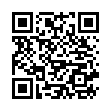# North Coast Synthesis Ltd.

## E♭♭5+2+4Choose audio sample set:
MSK 007 Leapfrog VCF (patch; see in online store)
MSK 009 Coiler VCF (patch; see in online store)

Equivalent chord symbols: F9-5+♯2, D♯♭5+2+4, D♯♭5+4+9, E♭♭5+4+9, D♯♭5+2+11, E♭♭5+2+11.

Notes in this chord: E♭, F, G, A♭, B♭♭. Integer notation: {3, 5, 7, 8, 9}.

Nearby chords (one less note): E♭4+2, E♭♭5+2, E♭♭5+4, G2+7+♯1, E♭sus4♭5+2.

Nearby chords (one more note): F9+♯2, E♭11♭5, E♭M11♭5, G9♯5+♯1, E♭+2+4+♯4, E♭♭5+2+4+♯1, E♭dim+2+4+♯3.

Parallel chords (same structure, different root): C♭♭5+2+4, D♭♭5+2+4, F♭♭5+2+4, G♭♭5+2+4, A♭♭5+2+4, B♭♭5+2+4, C♯♭5+2+4, D♯♭5+2+4, E♯♭5+2+4, F♯♭5+2+4, G♯♭5+2+4, A♯♭5+2+4, B♯♭5+2+4.

Experimental fretting charts for guitar standard EADGBE tuning (change tuning or instrument):